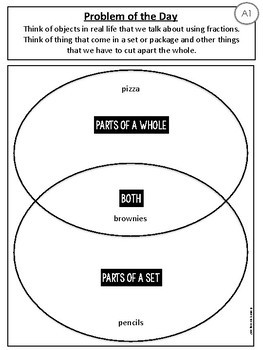# Differentiated Daily Math: FractionsSubject
Resource Type
File Type
PDF (9 MB|129 pages)
Standards
\$5.50
Also included in:
1. Get all of my Differentiated Daily Math pages in this one-stop bundle. With this purchase you will get all Differentiated Daily Math Units. These units are ideal for grades 4 & 5 classrooms. They allow you to differentiate for students who are working within grade expectations for grades 3-6.
\$74.00
\$64.95
Save \$9.05
• Product Description
• Standards
Teach all of your students and differentiate easily with these differentiated daily math pages. These pages will allow you to use one page and differentiate it is many different ways to meet a variety of needs in your classroom.

Curriculum Standards
- Meets the Ontario Curriculum expectations for grades 3-6
- Meets Common Core State Standards 3rd and 4th Grade

Fraction Math Skills Covered
- Fractions of a whole
- Fractions of a set
- Divide whole objects into equal parts
- Use fractional names
- Represent, compare and order fractions with like and unlike denominators
- Equivalent fractions

FAQ
What do you mean diffferentiated?
These are differentiated because you can use one page for every student. This means that your students working with below grade level expectations or above will also do the same pages. They are differentiated because the questions are designed to increase or decrease the complexity based on the fraction you start with.

How does it work?
Each day you will give students one double sided page. Page one includes familiar practice pages. This page is repeated and familiar each day. However the work each day is different. As you work through this front page the questions become familiar and routine as students master these skills through routine and practice. On page two the there is a problem of the day. This problem can be used as a modelled lesson, a partner activity, the basis for your number talks, or quick assessments.

If everyone is doing something different isn't it more work?
Actually it is less!! There is less work because you have less prep. By grouping students you can give each group a different starting point each day. They use their numbers to show mastery. Because the pages are familiar it is easy to track growth and understanding quickly as students are working.
Solve word problems involving addition and subtraction of fractions referring to the same whole and having like denominators, e.g., by using visual fraction models and equations to represent the problem.
Compare two fractions with different numerators and different denominators, e.g., by creating common denominators or numerators, or by comparing to a benchmark fraction such as 1/2. Recognize that comparisons are valid only when the two fractions refer to the same whole. Record the results of comparisons with symbols >, =, or <, and justify the conclusions, e.g., by using a visual fraction model.
Explain why a fraction 𝘢/𝘣 is equivalent to a fraction (𝘯 × 𝘢)/(𝘯 × 𝘣) by using visual fraction models, with attention to how the number and size of the parts differ even though the two fractions themselves are the same size. Use this principle to recognize and generate equivalent fractions.
Explain equivalence of fractions in special cases, and compare fractions by reasoning about their size.
Understand a fraction as a number on the number line; represent fractions on a number line diagram.
Total Pages
129 pages